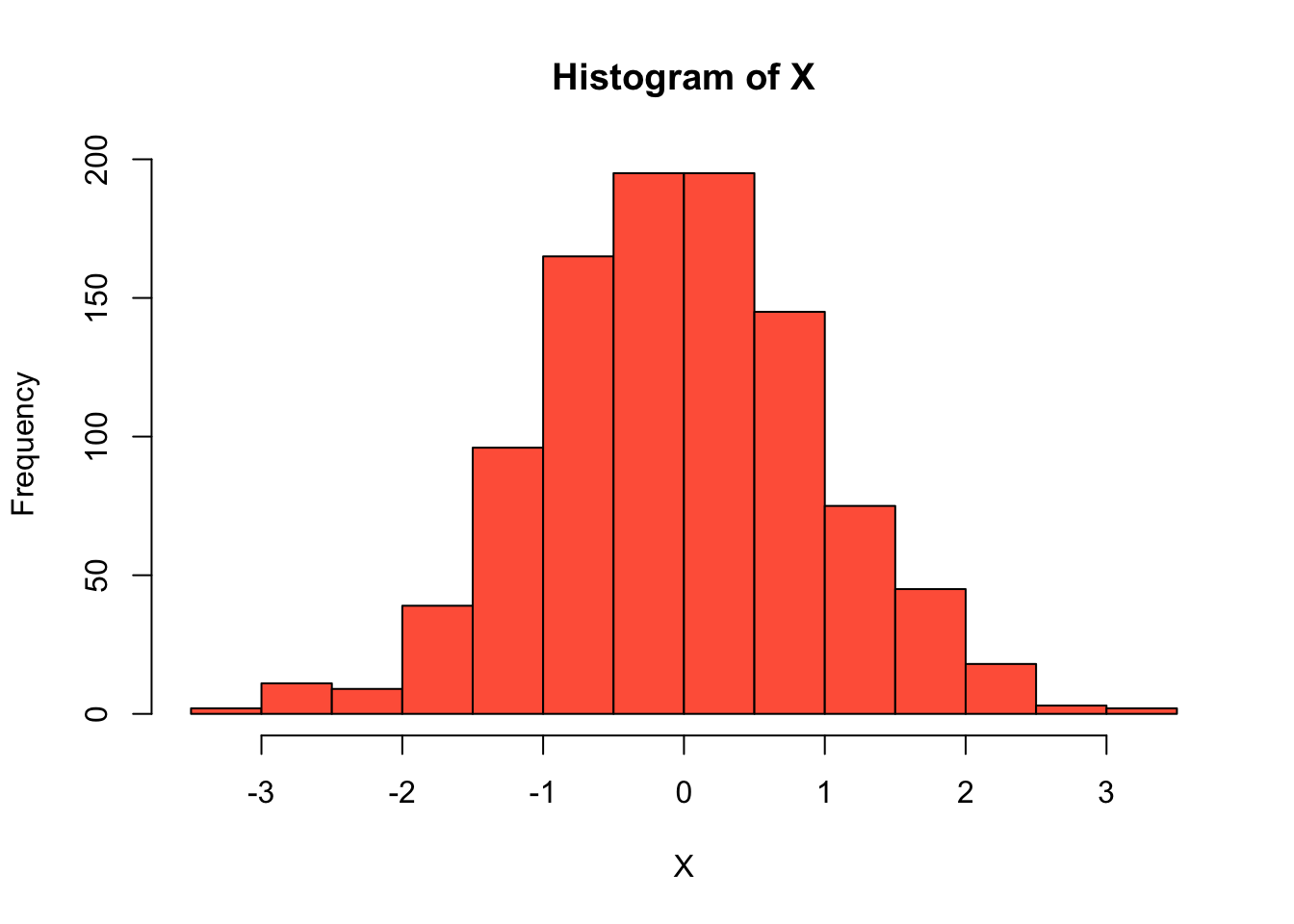### Random normal variables in R

If you worked with random variables before, in classical probability theory courses that do not require any statistical software, you might have used special tables to calculate probabilities for standard normal variables or binomial ones. Actually, in real research people rarely do that because it is possible to compute anything in R, Python, STATA or using a scientific calculator.

Let’s start from generation of random variables in R. To be more precise, all random values generated by computers are not purely random, they are pseudorandom since algorithms used for generation are deterministic, so numbers obtained are predictable.

Now we will create a sample of 1000 observations taken from a standard normal distribution:

# rnorm - random normal
# mean is the expected value (mu)
# sd is the standard deviation (sigma)
X <- rnorm(1000, mean = 0, sd = 1)
head(X)
##  -0.83572623  0.01572484  1.17525766  0.70531399 -0.46586740  0.53242011

As X is a random sample, its values will be different for all people running the code above. Moreover, they will be different if we repeat the operation:

X <- rnorm(1000, mean = 0, sd = 1)
head(X)
##  -0.49180685 -0.72384646  1.52833831  0.15106414 -0.73146989  0.04735057

Sometimes it is not important, but if our research includes computer simulations that have to be reproducible, we should make sure that all users will get the same results. To do so, we need the set.seed() function that specifies the starting point of a random algorithm making the output “frozen”:

set.seed(1234)
X <- rnorm(1000, mean = 0, sd = 1)
head(X)
##  -1.2070657  0.2774292  1.0844412 -2.3456977  0.4291247  0.5060559

And repeat with the seed set:

set.seed(1234)
X <- rnorm(1000, mean = 0, sd = 1)
head(X) # the same!
##  -1.2070657  0.2774292  1.0844412 -2.3456977  0.4291247  0.5060559

Let’s plot a histogram and look at the shape of X distribution:

hist(X, col="tomato")  # bell-shaped, normalThe rnorm() is one of the functions used to handle normal variables. If we ask R for help, we will see a detailed documentation for all functions:

help(rnorm)

The most useful are pnorm() and qnorm(). The former calculates the probabilities like $$P(X<a)$$ for $$X \sim N(\mu, \sigma^2)$$ and the latter calculates the quantiles of a certain level $$p$$, so finds $$x$$ such that $$P(X<x)=p$$.

Consider the following example. We know that $$X \sim N(\mu = 5, \sigma^2 = 9)$$ or $$X \sim N(\mu = 5, \sigma = 3)$$ and we are interested in $$P(X < 2)$$. Let’s compute it using R:

pnorm(2, mean = 5, sd = 3)  # ok
##  0.1586553

We can find the quantile of the level 0.65, i.e. the value $$x$$ of $$X$$ such that $$P(X<x) = 0.65$$.

qnorm(0.65, mean = 5, sd = 3)
##  6.155961

Now we will use this function to show that the limits of typical values of a variable calculated for box plots approximately coincide with those obtained by the three-sigma rule. Recall: box plots are informative for variables whose distributions are normal or close to normal (not seriously skewed).

Let us take a standard normal variable (default one in norm-functions in R) and calculate its lower and upper quartiles:

q1 <- qnorm(0.25)
q3 <- qnorm(0.75)
cat(q1, q3)
## -0.6744898 0.6744898

And the interquartile range:

iqr <- qnorm(0.75) - qnorm(0.25)
iqr
##  1.34898

Now compute two endpoints: [$$Q_1-1.5*IQR$$; $$Q_3+1.5*IQR$$].

q1 - 1.5 * iqr
##  -2.697959
q3 + 1.5 * iqr
##  2.697959

These values (-2.7 and 2.7 approximately) are close to -3 and 3 that we get applying the three-sigma rule. Recall: the three-sigma rule states, in particular, that 99% of values of a normal variable lie between [$$\mu-3\sigma$$; $$\mu+3\sigma$$], so here we get [$$0-3\cdot 1$$; $$0 + 3\cdot 1$$].

To see the same fact in a more interesting way, see the visualization in Wikipedia.